Mobile QR Code1. (Department of Electrical and Computer Engineering, Seoul National University, 1 Gwanak-ro, Gwanak-gu, Seoul 08826, Korea)

CMOS, nanoelectromechanical (NEM) memory switch, monolithic three-dimensional (M3D) integration, field programmable gate array (FPGA)

## I. INTRODUCTION

Semiconductor technology, which has brought about innovation in the information age, is developing day by day. Technologies that existed only as science fiction until just 10 years ago have been realized thanks to the development of semiconductors, and the era of intelligent revolution has arrived, where vast amounts of information can be retrieved within a few efforts. However, as the technology progresses, the ultra-low power consumption level required in the industry is starting to face a limit that cannot be achieved with modern silicon-based CMOS semiconductor devices. A representative of them is that the performance of hardware based on complementary metal-oxide-semiconductor (CMOS) is gradually slowing down to meet the standards required by the software industry. Therefore, it is necessary to develop a new concept semiconductor device that overcomes the limitations of existing technologies based on a new structure and operating principle. As can be seen in Fig. 1, in the case of a field-programmable gate array (FPGA) made of CMOS, most of the part that plays the role of routing rather than the part in charge of calculation is the root cause of this problem. From this components, leakage power and signal delay are occurring, and it can be also said that these routing devices cause loss in the overall chip integration .

##### Fig. 1. Distribution of area, signal delay, and leakage power of CMOS-based FPGAs.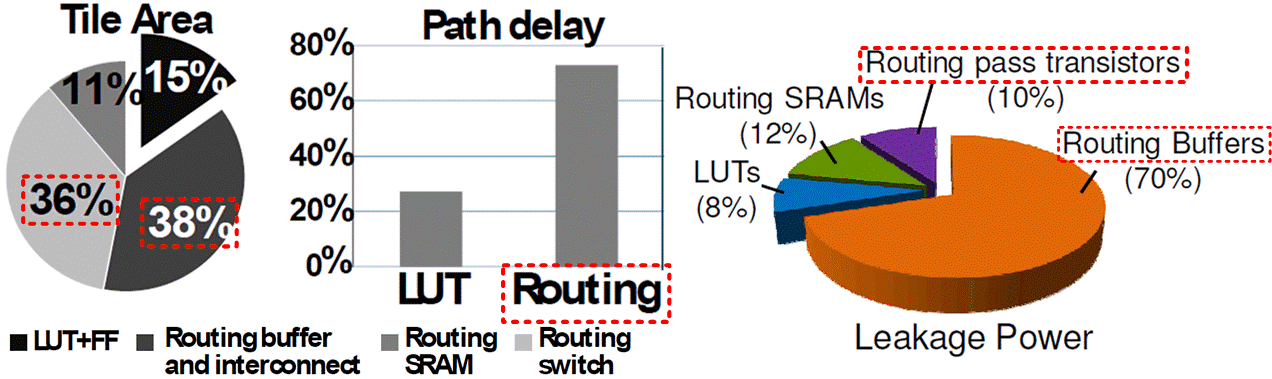To deal with aforementioned issues, CMOS-NEM RL circuits have been proposed that use CMOS baseline circuits for logic operation and NEM devices for routing operation, which are expected to save 37% leakage power, 28% signal delay and 43% area compared with conventional CMOS-only ones [1-12]. Fig. 2 schematically describes the structural and performance advantages of CMOS-NEM heterogeneous integrated circuits. Unlike conventional CMOS-based FPGA, proposed heterogeneous integrated circuit uses an NEM memory switch that makes the data signal path have non-volatile characteristics as a device in charge of routing.

##### Fig. 2. Structural schematic diagram of CMOS-NEM RL chip.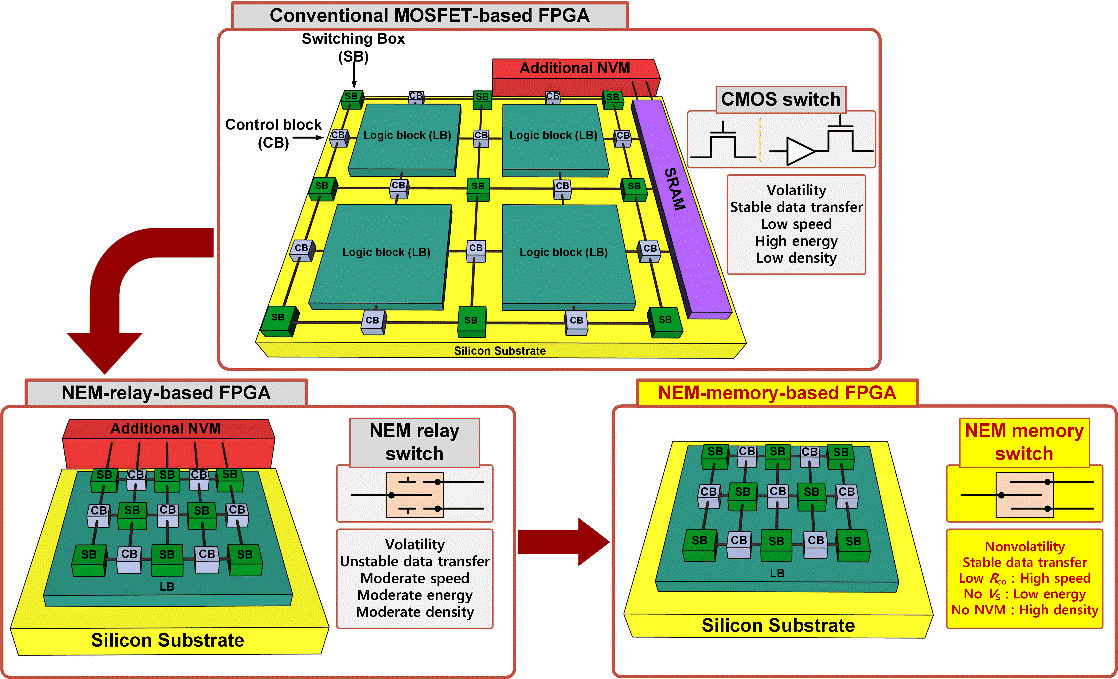In this manuscript, simulation and experimental demonstration of 65-nm process based M3D CMOS-NEM RL circuits were proposed which its schematic concept is shown in Fig. 3(a). Unlike conventional CMOS-only RL circuits where logic blocks (LBs), switch blocks (SBs), and connection blocks (CBs) consist of CMOS devices on a silicon substrate, as shown in Fig. 3(b), proposed M3D CMOS-NEM RL circuits replace CMOS-based SBs and CBs with NEM-based ones integrated on metal layers, as shown in Fig. 3(c).

##### Fig. 3. (a) Schematic view of the island-style RL structure representing single-tile and tile-to-tile operation. Conceptual view of (b) conventional CMOS only FPGA structure; (c) proposed CMOS-NEM integrated FPGA structure.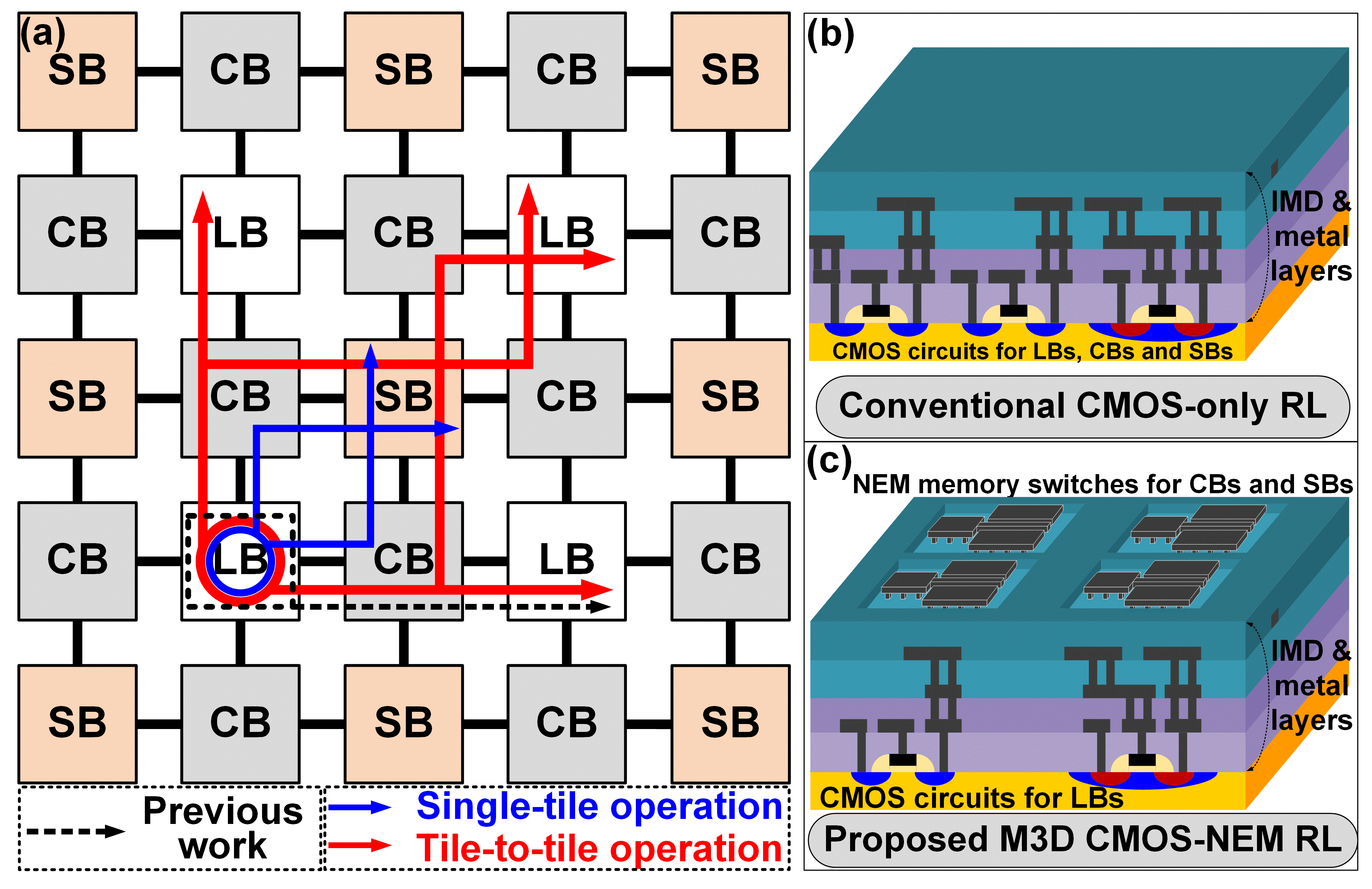## II. RESEARCH TRENDS OF NEM DEVICES

Brief research trends of NEM memory devices moved on from 2D integration to monolithic 3D integration as shown in Fig. 4. Before NEM devices were taken into the CMOS integration field, general trends were to facilitate a variety of functions in a single NEM device [13-15]. There was a variety of attempt to improve the efficiency of a single NEM device. To introduce some cases, in 2010, novel dual-ended (seesaw) structure NEM relay was demonstrated  showing the functionality of two relays with a single movable structure. Moving onto the nonvolatile NEM memory device, in 2010, H-cell structure that can store 4 bits in one cell was demonstrated . And in 2011, T-cell structure that can store 2 bits in one cell was demonstrated . Still, this was the era when full-scale CMOS-NEM heterogeneous integration was not demonstrated yet.

##### Fig. 4. Brief research trends of NEM devices.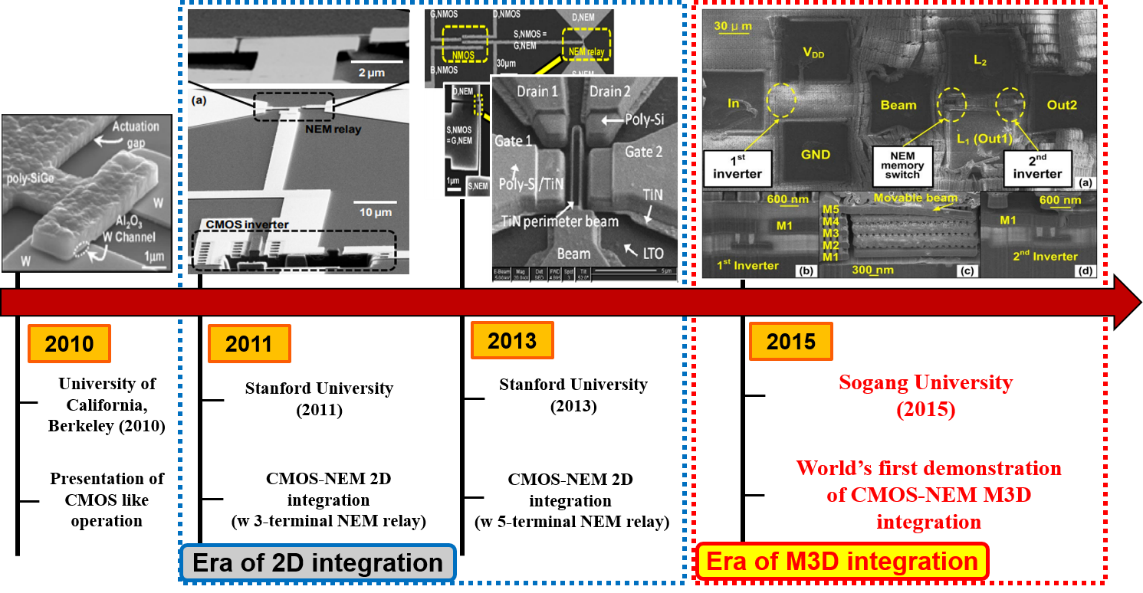After the diverse demonstration of single NEM devices were brought to the academia, research results of 2D integration of CMOS and NEM devices on a silicon substrate started to be published. Stanford's research team has published the results of 2D integration of simple CMOS-NEM RL which integrated each inverter and nMOS with NEM relay . However, 2D integration had a clear disadvantage in terms of chip density because the NEM device was integrated on a same layer with CMOS, which had a relatively large area compared to CMOS logic circuits.

Finally, the era of monolithic 3D (M3D) integration came to the academia by our group’s research in 2015 . This work was highly suggestive as the first case of heterogeneous integration of NEM devices in three dimensions on the upper metal layer without encroaching on the space of the CMOS baseline circuit. By opening the field of monolithic 3D integration, much more flexible signal path configuration is possible in CBs and SBs for routing. Furthermore, research on integrating NEM devices of various structures with CMOS is being actively conducted.

The current interest in M3D CMOS-NEM RL circuit can be focused in the following topics: Operating voltage (switching voltage of the NEM device), power consumption, speed and chip density. Since NEM switches has continuously suffered from their high operating voltages and low endurance cycles, most previous experimental results demonstrated the case in which the operating voltage of NEM switches exceeds the operating voltage (V$_{\mathrm{DD}}$) of CMOS devices [2, 4, 10]. This is a problematic issue because if the operating voltage (Switching voltage) of NEM routing switches is higher than the V$_{\mathrm{DD}}$ value of CMOS-only circuits, additional high-voltage charge pump circuits need to be introduced  and the system reliability will be degraded due to CMOS logic gate dielectric breakdown. Therefore, the operating voltage of the NEM memory switch has to scale down with the V$_{\mathrm{DD}}$ downscaling of the CMOS devices. To lower the operating voltage of the NEM memory device, one of the simplest answer is to scale down the dimension of the beam (especially the gap between the beam and electrode) .

Also, low endurance cycle has been an issue of implementing NEM memory device in the FPGA operation. According to the previous study on FPGA routing element, for the generic FPGA applications of NEM memory switches, \textasciitilde{}500 switching cycle number is known to be more than sufficient'' . However, various researches is still suffering from this issue because the demonstrated endurance cycle was far from acting as a routing device in FPGA applications. For example, S. Axelsson et al. demonstrated one switching cycle of gold NEM relays in 2005 , Y. Hayamizu et al. demonstrated 10 switching cycles of CNT NEM devices in 2008  and L. P. Tatum et al. demonstrated three switching cycles of copper NEM memory switches . Therefore, increasing the maximum number of endurance cycle is still considered as a challenge for FPGA applications of NEM devices.

In terms of power consumption and speed, previous simulation study investigating CMOS-NEM RL circuit exhibits the best performance overall regarding configuration, dynamic and stand-by mode . And for the chip density issues, with the downscaling of a single NEM memory area, integrating each NEM memory switch in a different metal layer is considered as a promising candidate as its concept is shown in Fig. 5. .

##### Fig. 5. Conceptual view of multi-layer NEM memory switches for enhanced chip density.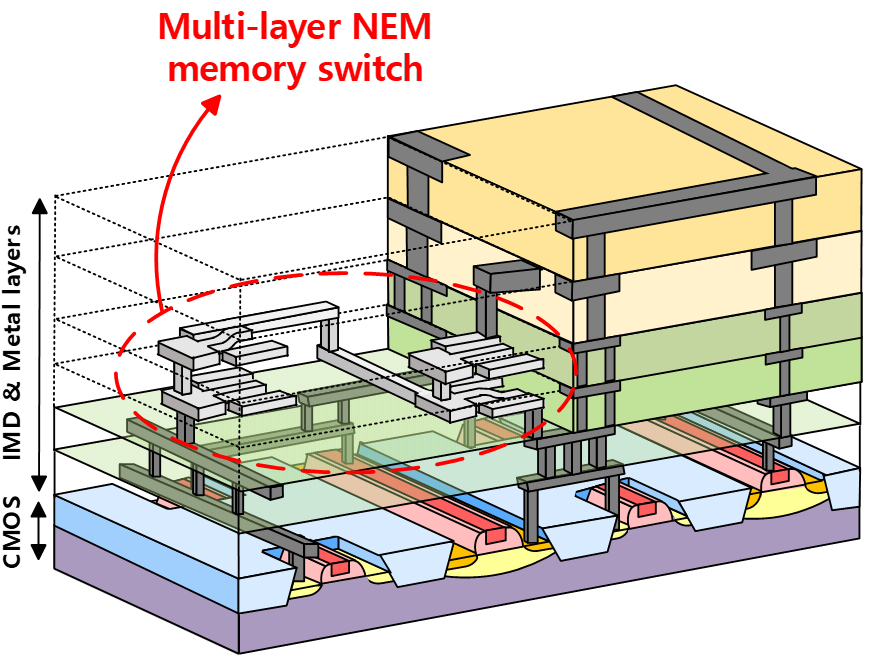## III. M3D CMOS-NEM RL CIRCUITS

### 1. NEM Device Configuration

The structure of the single NEM memory device is illustrated in Fig. 6. As shown in the figure, the designed NEM memory switch allows the beam to move in the lateral direction. Two electrodes, Line 1 and Line 2, exist in both sides of the beam, and the beam moves by the electrostatic force generated when voltage is applied to one of the two electrodes. When the voltage applied to the electrode is higher than the pull-in voltage (V$_{\mathrm{pull-in}}$), the beam is attached to the either side of the electrode (L1 or L2), which is called the turn-on state. It is designed to have non-volatile characteristics that can sustain each states thanks to the Van Der Waals force between the beam and electrode. That is, the stiction force, which is the attractive force that occurs between the beam and the electrode when the distance between the beam and the electrode is very close or when they are attached [22,23].

##### Fig. 6. Structure of a 3-terminal NEM memory device.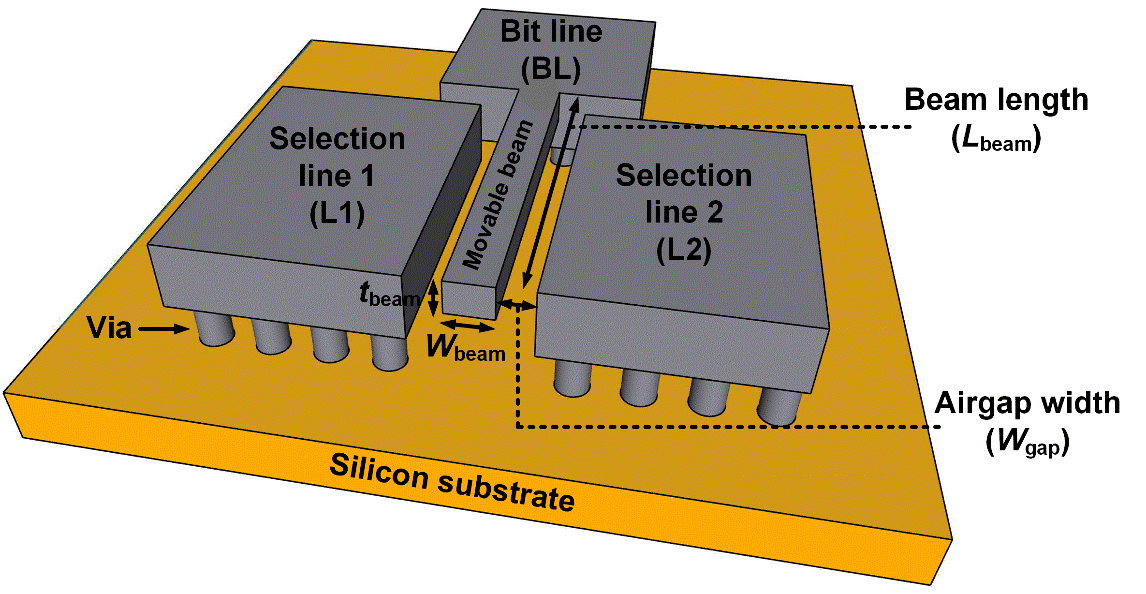The equivalent circuit model established for the cantilever structure is shown in Fig. 7. As shown in the figure, three states of NEM memory switch has different equivalent circuits. The parameters of the above equivalent circuit are as follows.

- R$_{\mathrm{BL}}$, R$_{\mathrm{L1}}$, R$_{\mathrm{L2}}$ : Metal line resistance

- R$_{\mathrm{CO}}$ : Contact resistance

-~C$_{\mathrm{gap1}}$, C$_{\mathrm{gap2}}$ : Capacitance between beam and electrode

Equations of the analytical model derived based on the above equation and the analysis results of the operation of the NEM device are as follows. Here, the term ‘x’ stands for the displacement of the beam. The beam position (x) is calculated by

##### (1)
$F_{\mathrm{elec}}=F_{\mathrm{ad}}-F_{\mathrm{r}}$

where F$_{\mathrm{elec}}$ means the electrostatic force between the beam and selection lines defined as

##### (2)
$F_{\text {elec }}=\left\{\begin{array}{l}\frac{\varepsilon_{0} L_{\text {beam }} W_{\text {beam }}\left(V_{\text {BL-L1 }}\right)^{2}}{2\left(t_{\text {gap1 }}-x\right)^{2}} \text { when } V_{\text {BL-L2 }}=0 \mathrm{~V} \\ \frac{\varepsilon_{0} L_{\text {beam }} W_{\text {beam }}\left(V_{\mathrm{BL}-\mathrm{L} 2}\right)^{2}}{2\left(t_{\text {gap1 }}-x\right)^{2}} \text { when } V_{\text {BL-L1 }}=0 \mathrm{~V}\end{array}\right.$

F$_{\mathrm{r}}$ means the restoring force of the beam defined as

##### (3)
$F_{\mathrm{r}}=\frac{2EW_{\mathrm{beam}}t_{\mathrm{beam}}^{3}}{3L_{\mathrm{beam}}^{3}}x$

(Case when 0 ${\leq}$ {\textbar}x{\textbar} ${\leq}$ t$_{\mathrm{gap1}}$ - d$_{\mathrm{vdw}}$, d$_{\mathrm{vdw}}$ is the Van Der Waals distance)

And F$_{\mathrm{ad}}$ means the van der Waals adhesion force between the beam and selection lines defined as

##### (4)
$F_{\text {ad }}= \begin{cases}\frac{2 A_{\text {TiN }} L_{\text {beam }} W_{\text {beam }} \alpha\left(d_{\text {vdw }}-\left(t_{\text {gap } 1}-x\right)\right)}{12 \pi D_{\text {mms }}^{2} d_{\text {vdw }}^{2}} & \text { when } t_{\text {gap } 1}-d_{\text {vdw }} \leq|\mathrm{x}| \leq t_{\text {gap } 1} \\ 0 & \text { when } 0 \leq|\mathrm{x}| \leq t_{\text {gap } 1}-d_{\text {vdw }}\end{cases}$
##### Fig. 7. Equivalent circuit of NEM switch with cantilever structure.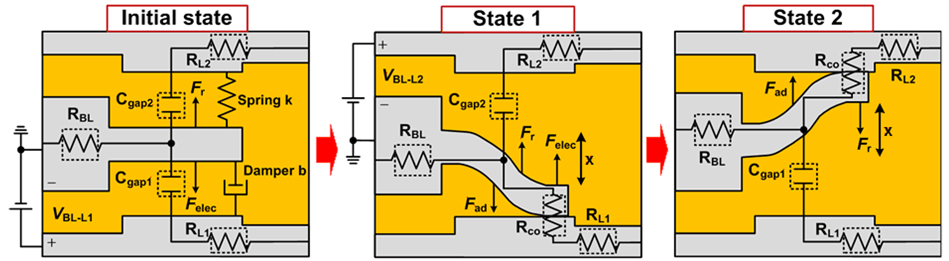Followed by the abovementioned equations, Fig. 8 shows the relationship between F$_{\mathrm{elec}}$, F$_{\mathrm{ad}}$ and F$_{\mathrm{r}}$ when the separation gap between the beam and selection lines is smaller than d$_{\mathrm{vdw}}$. If the beam is stuck to L1 and nonzero V$_{\mathrm{BL-}}$$_{L}$$_{2}$ is applied, F$_{\mathrm{elec}}$ is applied to move the beam to L2. If V$_{\mathrm{BL-L2}}$ is not high enough to move the beam, the NEM memory switch remains State 1 because the unstable solution of (1) exists within the air gap as shown in Fig. 9(b). On the other hand, if V$_{\mathrm{BL-L2}}$ is high enough to move the beam to L2, the beam is stuck to L2. It means that State 1 is changed into State 2 because the unstable solution of (1) exists out of the air gap .

##### Fig. 8. (a) Relationship between beam displacement and applied forces; (b) Relationship between VBL-L2 and beam displacement .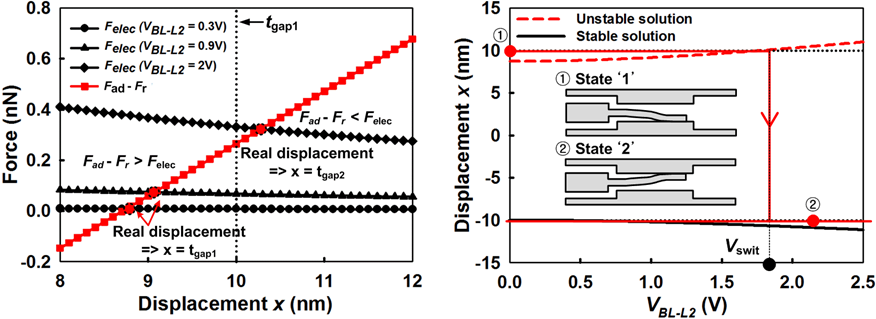##### Fig. 9. Key fabrication process of M3D CMOS-NEM RL circuit.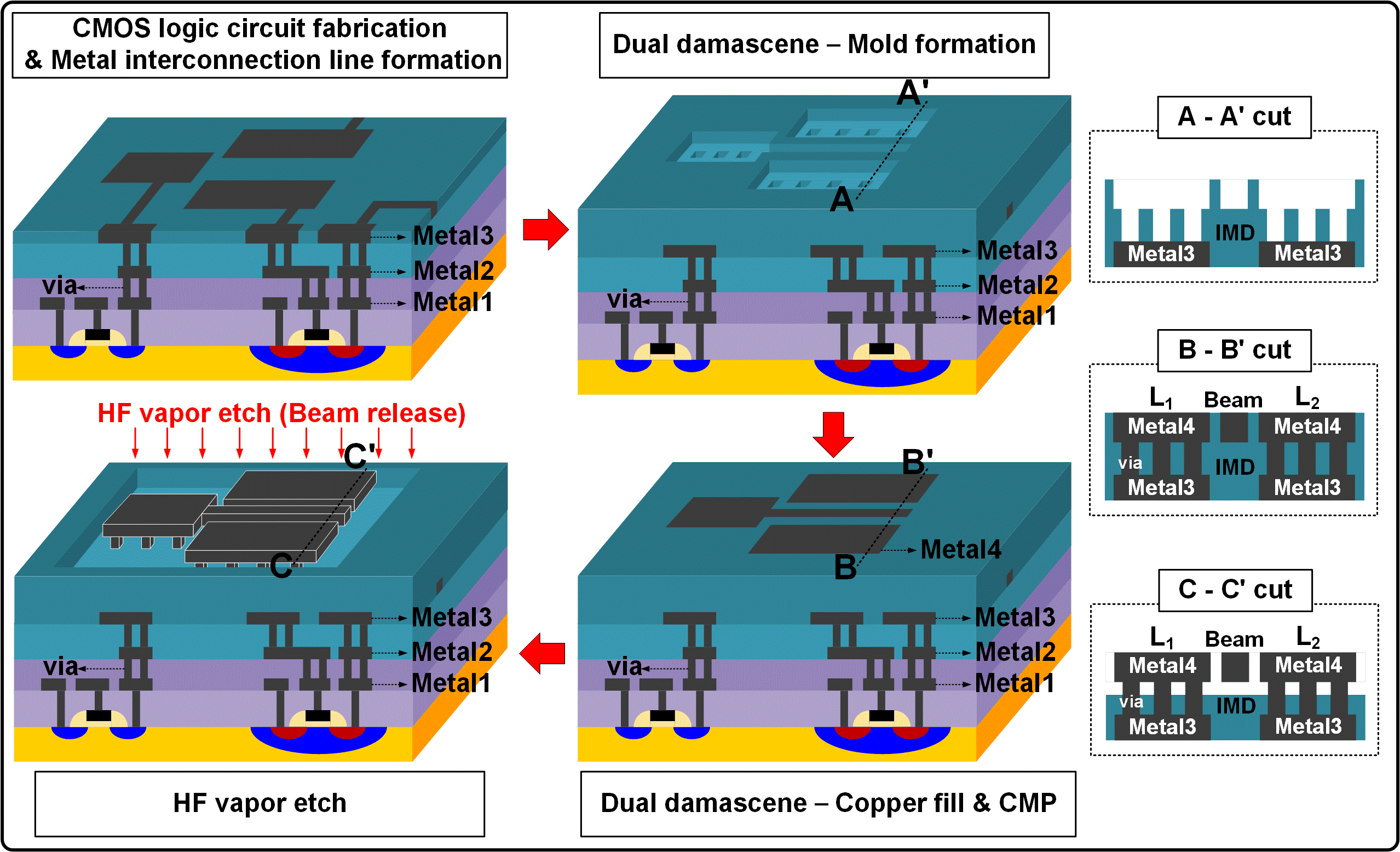### 2. Fabrication Process

Fig. 9 shows the key process steps of the proposed circuit. First, CMOS logic circuits are fabricated on a silicon substrate by using the standard 65-nm CMOS front-end-of-line (FEOL) process. Then, metal interconnection lines and vias are formed by using the standard CMOS back-end-of-line (BEOL) process. Subsequently, NEM memory switches are patterned by using conventional ArF. Because copper is introduced for interconnection lines and NEM memory switches, a dual damascene process has been used. In our previous work , aluminum was used for interconnection lines and NEM memory switches. In addition, NEM memory switches were patterned by a focused ion beam (FIB) process. Even though NEM memory switches can be formed in any metal interconnection layer, in this work, they are formed in the fourth metal layer for the convenience of HF vapor release process. Finally, the inter-metal dielectric (IMD) layers surrounding the NEM memory switches are selectively removed to release the movable beams of the NEM memory switches by using HF vapor etching at 40 $^{\circ}$C for 6 min. 49-% aqueous HF solution is used for this process. It should be noted that the entire fabrication process is identical to the 65-nm CMOS baseline process except for the HF vapor etching used to release the NEM memory switches.

Fig. 10 and 11 shows the schematic and SEM image of the fabricated M3D CMOS-NEM RL circuit. The dimensions of the NEM memory switch are summarized in Table 1. To be specific, Fig. 11(a) and (b) show the plan-view scanning electron microscopy (SEM) images of the fabricated M3D CMOS-NEM RL circuits that correspond to Fig. 11(a) and (b), respectively. Fig. 11(c) and (d) show the plan and cross-sectional view of the fabricated NEM memory switch. It is observed that NEM memory switches are placed in the fourth metal layer as shown in Fig. 11(d). It operates as a one-to-two multiplexer toggling between two output terminals. Logic and routing parts consist of CMOS and NEM memory devices, respectively. Data signal paths and logic functions vary as a function of the movable beam position of NEM memory switches depending on the WL and BL signals.

##### Fig. 10. Schematic of the M3D CMOS-NEM RL circuit for (a) single-tile operation; (b) tile-to-tile operation.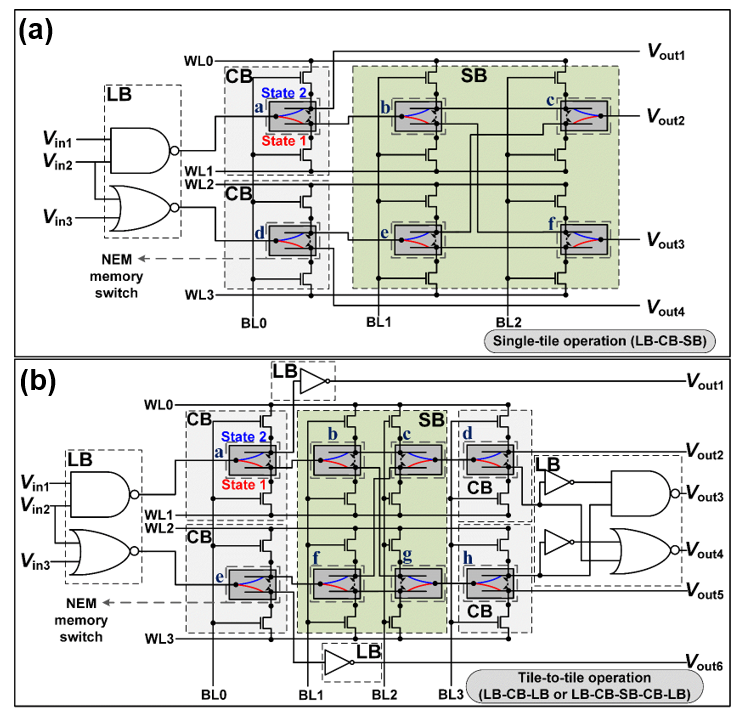##### Fig. 11. Plan view of the fabricated M3D CMOS-NEM RL circuit for (a) single-tile operation; (b) tile-to-tile operation; (c) Plan; (d) cross-sectional view of the fabricated NEM memory switch.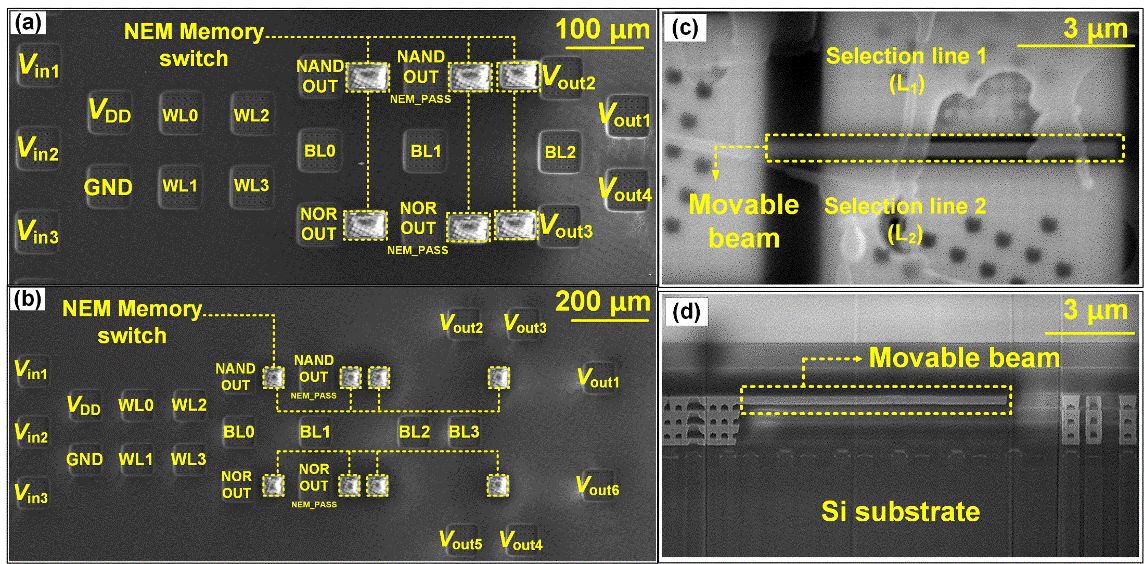##### Table 1. Design parameters of the fabricated NEM memory switch
 Movable beam length (L$_{\mathrm{beam}}$) 9.0 um Movable beam width (W$_{\mathrm{beam}}$) 220 nm Movable beam thickness (t$_{\mathrm{beam}}$) 180 nm Airgap widths (W$_{\mathrm{gap1,2}}$) 115 nm Movable beam material Copper

## IV. RESULTS AND DISCUSSION

In this section, simulation and measurement results of the M3D CMOS-NEM RL circuits are demonstrated. Simulation results were verified through H-Spice-based integrated circuit modeling. Even if the NEM memory device is integrated in the extended circuit, superior performance is guaranteed compared to the circuit constructed using only CMOS. The implementation of the NEM memory device in the circuit was performed using Verilog-A. The logic part of the circuit consists of NAND and NOR circuits in order to be able to check all outputs of each single tile and tile-to-tile operation.

In the case of tile-to-tile operation, an inverter was added to the logic circuit part to subdivide the output signal. The results of comparative analysis of the operating frequency, power consumption, and area consumption in the above circuit are shown in the Table 2. The simulation conducted to evaluate the advantages of M3D CMOS-NEM RL circuit was conducted based on the dynamic mode. This is because dynamic mode occupies the largest proportion compared to configuration mode or standby mode .

Circuit simulation was carried out regarding all combination cases (2$^{6}$ logic combinations for single tile and 2$^{8}$ logic combinations for tile-to-tile) and the power and energy consumption values in each case were extracted at 500 MHz reflecting the lowest operating frequency case (tile-to-tile, pass gate case). As a result of the simulation, proposed M3D CMOS-NEM RL circuit exhibit 6.6x and 4.6x higher chip density, 1.1x and 2.3x higher operation frequency and 55.0x and 9.3x lower power consumption than CMOS-only ones (tri-state buffer case) for single-tile and tile-to-tile operation, respectively. The comparison of area components was based on the layout which was used for actual process. The difference in case of pass gate versus NEM memory switch originates from the purpose of CMOS device used in each cases. In case of pass gates, multi finger CMOS devices were used in the circuit design because these circuits has to drive high current. But in case of access transistors for NEM memory switches, the purpose of access transistors are not to drive high current but to operate proper NEM memory switches based on the WL/BL signals. Therefore, it does not need to have wide width allowing to design it in a minimum channel width.

In other words, it was verified based on simulation that the heterogeneous integrated circuit proposed by this work team has advantages over existing circuits even in extended application circuits rather than circuits composed of single elements.

From now, the measurement data of the fabricated M3D CMOS-NEM RL circuit will be presented. Fig. 12 shows the current-vs.-voltage curves of the fabricated NEM memory switch. It is observed that the switching voltage of NEM memory switches increases as switching cycles are repeated. When NEM memory switches are toggled between States 1 and 2, maximum stress is applied to the connecting region between the movable beam and anchor pad. Thus, repeated switching operations make the movable beam less elastic, which increases the switching voltage.

Fig. 13 and 14 shows the measurement results of the M3D CMOS-NEM RL circuits for single-tile and tile-to-tile operation, respectively. Among the 2$^{6}$ or 2$^{8}$ logic combinations of single-tile or tile-to-tile operation, several exemplary cases are presented. Input signals (V$_{\mathrm{in1}}$, V$_{\mathrm{in2}}$, and V$_{\mathrm{in3}}$) are the square waves whose magnitude and frequency are 1.2 V and 500 KHz, respectively. Signal paths are changed following the state of NEM memory switches. The noise of the measurement results stems from the parasitic capacitance of large measurement pads and measurement equipment.

In the case of single-tile operation, as shown in Fig. 13(a) and (b), the outputs of the RL circuit consist of NAND (/(V$_{\mathrm{in1}}$*V$_{\mathrm{in2}}$)) and NOR (/(V$_{\mathrm{in2}}$+V$_{\mathrm{in3}}$)) logic functions. V$_{\mathrm{out1}}$ and V$_{\mathrm{out4}}$ can print only one of them while V$_{\mathrm{out2}}$ and V$_{\mathrm{out3}}$ can print both of them depending on the signal paths generated by the NEM memory switches in CB and SB.

On the contrary, in the tile-to-tile operation, additional inverters are added to the second stage of the LB to accurately verify the source of the output signal owing to the complex signal paths composed by the states of the CBs and SBs. As shown in Fig. 14(a) and (b), V$_{\mathrm{out1}}$ and V$_{\mathrm{out6}}$ can print AND (V$_{\mathrm{in1}}$*V$_{\mathrm{in2}}$) and OR (V$_{\mathrm{in2}}$+V$_{\mathrm{in3}}$) logic functions, respectively. Additionally, as shown in Fig. 14(c) and (d), V$_{\mathrm{out2}}$ and V$_{\mathrm{out5}}$ can print either NAND (/(V$_{\mathrm{in1}}$*V$_{\mathrm{in2}}$)) or NOR (/(V$_{\mathrm{in2}}$+V$_{\mathrm{in3}}$)) logic functions, respectively. Moreover, as shown in Fig. 14(e) and (f), V$_{\mathrm{out3}}$ can print either /((V$_{\mathrm{in1}}$*V$_{\mathrm{in2}}$)*/(V$_{\mathrm{in2}}$+V$_{\mathrm{in3}}$)) or /((V$_{\mathrm{in2}}$+V$_{\mathrm{in3}}$)*/(V$_{\mathrm{in1}}$*V$_{\mathrm{in2}}$)) because it is a combination of the inverted output of V$_{\mathrm{out2}}$ and the output of V$_{\mathrm{out5}}$ passing through the NAND gate. Meanwhile, the output of signal V$_{\mathrm{out4}}$ is a combination of the inverted output of V$_{\mathrm{out5}}$ and the output of V$_{\mathrm{out2}}$ passing through the NOR gate. Thus, as shown in Fig. 14(g) and (h), V$_{\mathrm{out4}}$ can print out either /(/(V$_{\mathrm{in1}}$*V$_{\mathrm{in2}}$)+(V$_{\mathrm{in2}}$+V$_{\mathrm{in3}}$)) or /(/(V$_{\mathrm{in2}}$+V$_{\mathrm{in3}}$)+(V$_{\mathrm{in1}}$*V$_{\mathrm{in2}}$)).

It is noteworthy that the fabricated CMOS inverters, and NAND and NOR logic gates have large gate delays that render the measured operating frequency significantly lower than our simulated values shown in Table 2. The difference between experimental and simulation data stems from the parasitic capacitance of large measurement pads and measurement equipment, which will be minimized in the commercialized version.

##### Table 2. Simulated characteristics of conventional CMOS-only and proposed M3D CMOS-NEM RL circuits
 Operation Single-tile operation Tile-to-tile operation RL circuit M3D CMOS-NEM (this work) CMOS-only M3D CMOS-NEM (this work) CMOS-only Pass gate Transmission gate Tri-state buffer Pass gate Transmission gate Tri-state buffer Max. freq. (GHz) 10.36 3.17 1.41 9.12 3.29 0.63 0.81 1.44 Power (${\mu}$W) 3.42 5.26 10.52 188.78 82.81 323.58 175.03 752.42 Area (F$^{2}$) 480 1632 2784 3168 992 2528 4064 4576
##### Fig. 12. Current vs. voltage curves of the fabricated NEM memory switch.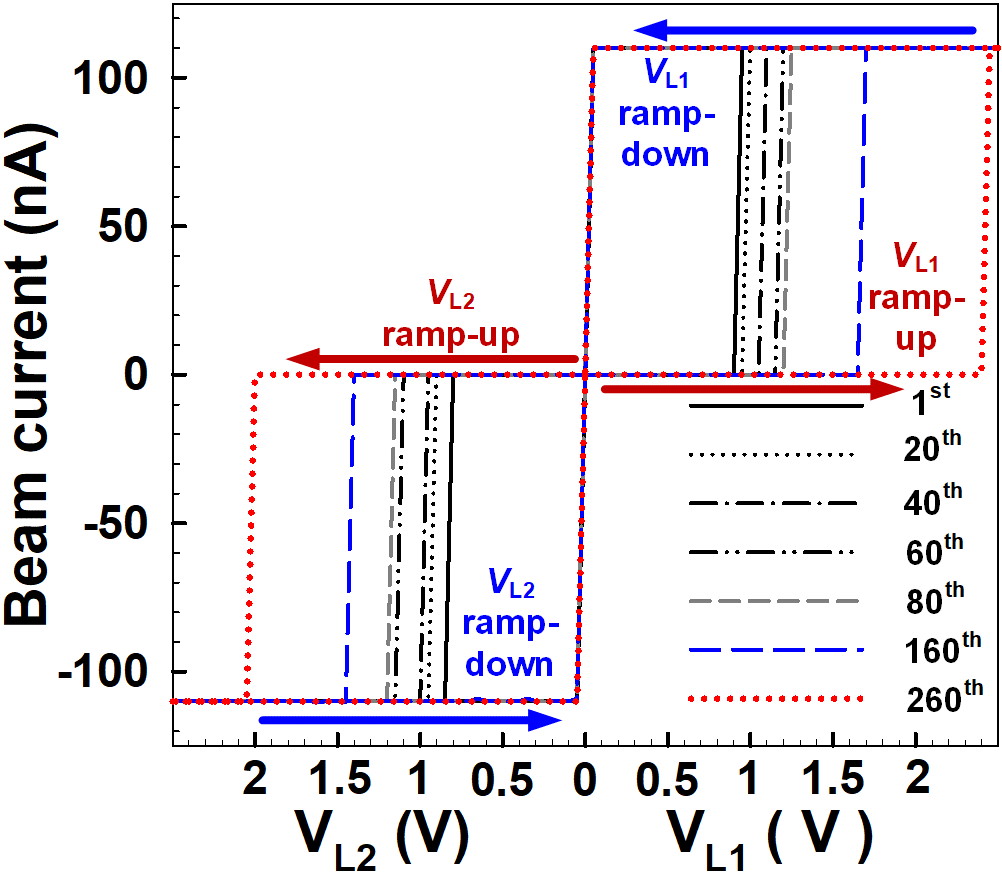##### Fig. 13. Measured input and output signals of the fabricated M3D CMOS-NEM RL circuit for single-tile operation: (a) NAND output case; (b) NOR output case.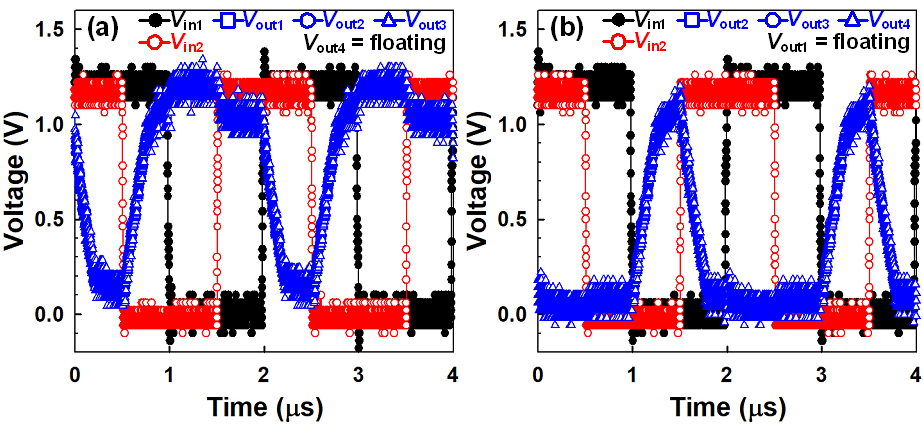##### Fig. 14. Measured input and output signals of the fabricated M3D CMOS-NEM RL circuit for tile-to-tile operation: (a) V$_{\mathrm{in1}}$*V$_{\mathrm{in2}}$ output case; (b) V$_{\mathrm{in2}}$+V$_{\mathrm{in3}}$ output case; (c) NAND output case; (d) NOR output case; (e) /((V$_{\mathrm{in1}}$*V$_{\mathrm{in2}}$) * /(V$_{\mathrm{in2}}$+V$_{\mathrm{in3}}$)) output case; (f) /((V$_{\mathrm{in2}}$+V$_{\mathrm{in3}}$) * /(V$_{\mathrm{in1}}$*V$_{\mathrm{in2}}$)) output case; (g) /(/(V$_{\mathrm{in1}}$*V$_{\mathrm{in2}}$) + (V$_{\mathrm{in2}}$+V$_{\mathrm{in3}}$)) output case; (h) /(/(V$_{\mathrm{in2}}$+V$_{\mathrm{in3}}$) + (V$_{\mathrm{in1}}$*V$_{\mathrm{in2}}$)) output case.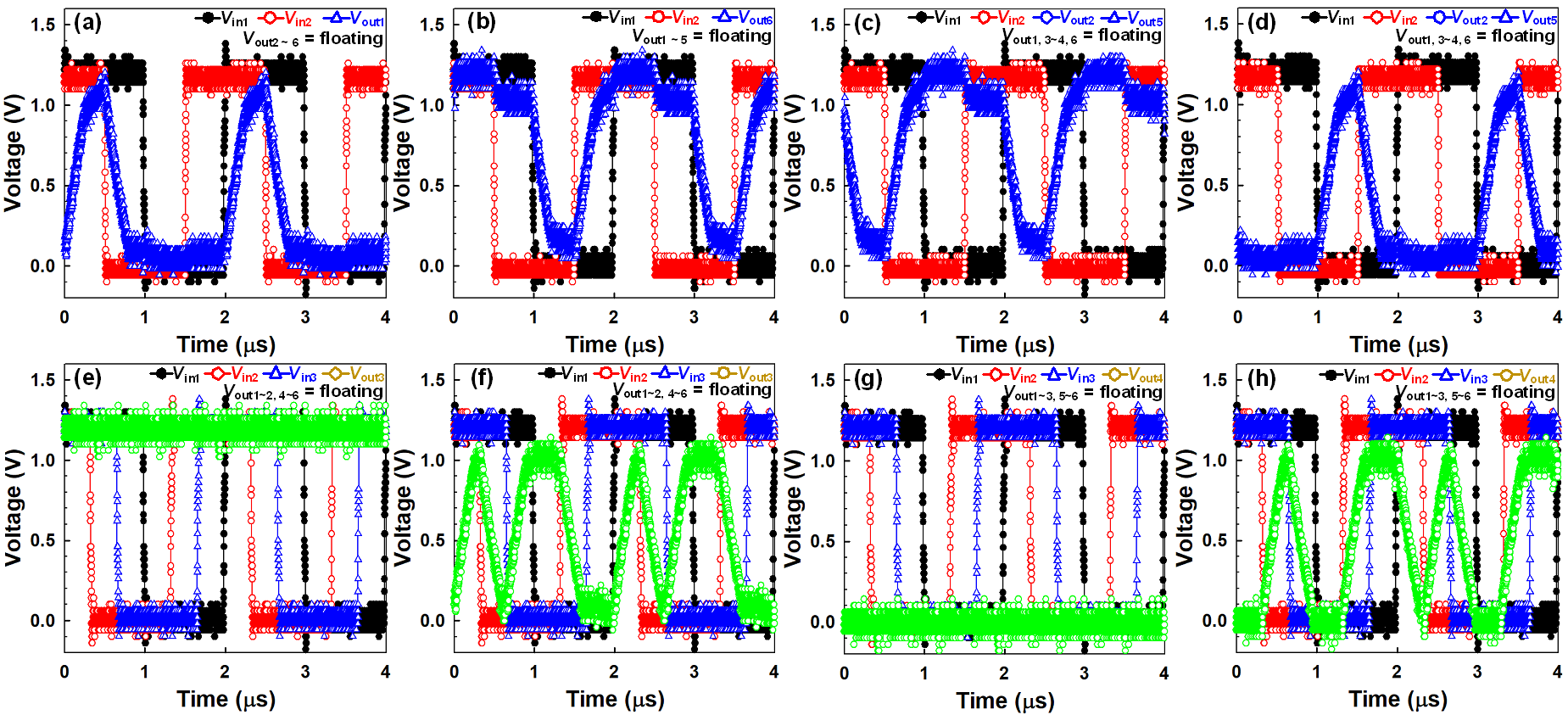## V. CONCLUSION

Recent studies on NEM devices for logic and memory applications has been reviewed from the perspective of M3D heterogeneous integration. To add to the prospects for the presented research, suggested CMOS-NEM RL circuit in this manuscript can improve the performance of integrated circuits without the continuous downscaling of existing CMOS devices. In addition, it is noteworthy that the BEOL integrated device technology is compatible with existing CMOS process. The feasibility of M3D CMOS-NEM RL circuits has been confirmed experimentally by implementing a single-tile and tile-to-tile RL operation. It was observed that the data signals processed by an LB are transferred to the following LBs successfully through the signal paths determined by NEM memory switches. It is expected that proposed M3D CMOS-NEM RL circuits will be expanded to implement FPGAs that are as fast as ASICs with low fabrication cost and short development time.

## ACKNOWLEDGMENTS

This work was supported by the NRF of Korea funded by the MSIT under Grant NRF-2021M3F3A2A01037927, NRF-2022M3F3A2A01073944 (Intelligent Semiconductor Technology Development Program), NRF-2022M3I7A1078544 (PIM Semiconductor Technology Development Program), and NRF-2021R1A2C1007931 (Mid-Career Researcher Program).

## References

1
Chen C., Lee W. S., Parsa R., Chong S., Provine J., Watt J., Howe R. T., Wong H.-S. P., Mitra S., Mar. 2012, Nano-electro-mechanical relays for FPGA routing: experimental demonstration and a design technique, in Proc. Conference on Design, Automation and Test, pp. 1361-13662
Chong S., Lee B., Parizi K. B., Provine J., Mitra S., Howe R. T., Wong H.-S. P., Dec. 2011, Integration of nanoelectromechanical (NEM) relays with silicon CMOS with functional CMOS-NEM circuit, in Proc. Int. Electron Devices Meeting (IEDM), pp. 30.5.1-30.5.43
Choi W. Y., Kim Y. J., Sep. 2015, Three-dimensional integration of complementary metal-oxide-semiconductor-nanoelectromechanical hybrid reconfigurable circuits, IEEE Electron Device Letters, Vol. 36, No. 9, pp. 887-8894
Munoz-Gamarra J. L., Uranga A., Barniol N., Feb. 2016., CMOS-NEMS copper switches monolithically integrated using a 65 nm CMOS technology, Micromachines, Vol. 7, No. 25
Chen C., Parsa R., Patil N., Chong S., Akarvardar K., Provine J., Lewis D., Watt J., Howe R. T., Wong H.-S. P., Mitra S., Feb. 2010, Efficient FPGAs using nanoelectromechanical relays, in Proc. Int. Symp. on Field-Programmable Gate Arrays, pp. 273-2826
Dong C., Chen C., Mitra S., Chen D., Jun. 2011, Architecture and performance evaluation of 3D CMOS-NEM FPGA, in Proc. System Level Interconnect Prediction Workshop, pp. 1-87
Song S.-H., Chun K. C., Kim C. H., Aug. 2014, A bit-by-bit re-writable eflash in a generic 65 nm logic process for moderate-density nonvolatile memory applications, IEEE J. Solid-State Circuits, Vol. 49, No. 8, pp. 1861-18718
Kam H., King Liu T.-J., Stojanovic V., Markovic D., Alon E., Jan. 2011, Design, optimization, and scaling of MEM relays for ultra-low-power digital logic, IEEE Transactions on Electron Devices, Vol. 58, No. 1, pp. 236-2509
Choi W. Y., Osabe T., King Liu T.-J., Dec. 2008, Nano-electro-mechanical nonvolatile memory (NEMory) cell design and scaling, IEEE Trans. Electron Devices, Vol. 55, No. 12, pp. 3482-348810
Lee H. M., Choi W. Y., Feb. 2017, Switching voltage modeling of nano-electromechanical (NEM) memory switches, The 24th Korean Conference on Semiconductors (KCS), pp. 3211
Kim Y. J., Choi W. Y., Feb. 2015, Nonvolatile nanoelectromechanical memory switches for low-power and high-speed field-programmable gate arrays, IEEE Transactions on Electron Devices, Vol. 62, No. 2, pp. 673-67912
Kwon H. S., Kim S. K., Choi W. Y., Sep. 2017, Monolithic three-dimensional 65-nm CMOS-nanoelectromechanical reconfigurable logic for sub-1.2-V operation, IEEE Electron Device Letters, Vol. 38, No. 9, pp. 1317-132013
Jeon J., Pott V., Kam H., Nathanael R., Alon E., Liu T. K., April 2010, Perfectly Complementary Relay Design for Digital Logic Applications, IEEE Electron Device Letters, Vol. 31, No. 4, pp. 371-37314
Choi W. Y., Jan. 2010, Three-Dimensional Stackable Electromechanical Nonvolatile Memory Cell (H Cell) for Four-Bit Operation, IEEE Electron Device Letters, Vol. 31, No. 1, pp. 29-3115
Lee K., Choi W. Y., Apr. 2011, Nanoelectromechanical Memory Cell (T Cell) for Low-Cost Embedded Nonvolatile Memory Applications, IEEE Transactions on Electron Devices, Vol. 58, No. 4, pp. 1264-126716
Chong S., Lee B., Mitra S., Howe R. T., Wong. H. -S. P., Jan. 2012, Integration of Nanoelectromechanical Relays With Silicon nMOS, IEEE Transactions on Electron Devices, Vol. 59, No. 1, pp. 255-25817
Kuon I., Tessier R., Rose. J., 2007, FPGA architecture: Survey and challenges, foundations and trends r in electronic design automation., Foundations and Trends in Electronic Design Automation, Vol. 2, No. 2, pp. 135-25318
Axelsson S., Campbell E. E. B., Jonsson L. M., Kinaret J., Lee S. W., Park Y. W., Sveningsson. M., 2005, Theoretical and experimental investigations of three-terminal carbon nanotube relays, New Journal of Physics, Vol. 7, pp. 24519
Hayamizu Y., Yamada T., Mizuno K., Davis R. C., Futaba D. N., Yumura M., Hata. K., 2008, Integrated three-dimensional micro-electromechanical devices from processable carbon nanotube wafers., Nature Nanotechnology, Vol. 3, pp. 289-29420
Tatum L. P., Sikder U., Liu. T. -J. K., 2021, Design technology co-optimization for back-end-of-line nonvolatile NEM switch arrays, IEEE Transactions on Electron Devices, Vol. 68, No. 4, pp. 1471-147721
Yoon J., Kwon H. S., Choi W. Y., Jan. 2022, Multi-Layer Nanoelectromechanical (NEM) Memory Switches for Multi-Path Routing., IEEE Electron Device Letters, Vol. 43, No. 1, pp. 162-16522
Soon B. W., Ng E. J., Qian Y., Singh N., Tsai M. J., Lee C., Aug. 2013, A bi-stable nanoelectromechanical nonvolatile memory based on van der Waals force, Applied Physics. Letter., Vol. 103, No. 5, pp. 053122-05312223
DelRio F. W., De Boer M. P., Knapp J. A., Reedy E. D., Clews Jr., P. J., Dunn M. L., Aug. 2005, The role of van der Waals forces in adhesion of micromachined surfaces, Nature Materials., Vol. 4, No. 8, pp. 629-634Hyug Su Kwon was born in Daejeon, in 1988. He received the B.S. and Ph. D. degrees in 2014 and 2022 in the Department of Electronic Engi-neering from Sogang University, Seoul, Korea. Since 2022, he is with the Department of Department of Electrical and Computer Engineering, Seoul National University (Seoul, Korea), as a post-doctor. His current research interests include nanoelectromechanical (NEM) devices, monolithic 3D (M3D) heterogeneous integration and 3D NAND flash memory devices.

Woo Young Choi (Senior Member, IEEE) received the B.S., M.S., and Ph.D. degrees from the School of Electrical Engineering, Seoul National University, Seoul, South Korea, in 2000, 2002, and 2006, respectively. From 2006 to 2008, he held a postdoctoral position with the Department of Electrical Engineering and Computer Sciences, University of California at Berkeley, Berkeley, CA, USA. From 2008 to 2022, he was a professor at the Department of Electronic Engineering, Sogang University, Seoul. Since 2022, he has been a Faculty Member with Seoul National University, Seoul, where he is currently an Associate Professor with the Department of Electrical and Computer Engineering. He has authored or coauthored more than 300 articles in international journals and conference proceedings. He holds more than 55 Korean/U.S. patents. His current research interests include fabrication, modeling, characterization, and measurement of CMOS logic/analog devices, emerging devices, memory devices, and brain-inspired computing devices.